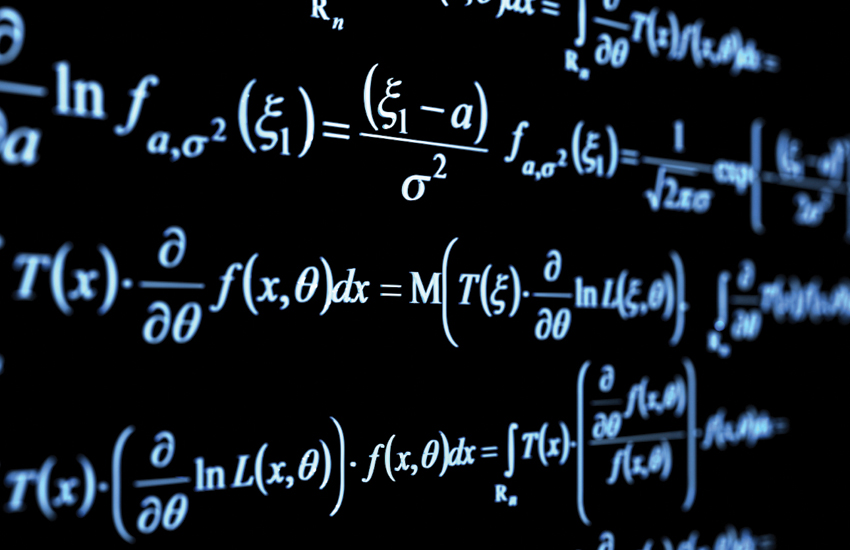Further MathsFurther Mathematics allows the study of a wider range of concepts and techniques in pure, mechanics and decision mathematics.  You focus on solving abstract problems and applying practical methods in the areas of engineering, computing and project management.

As it is an advanced area of study you will need to be very interested in mathematics to consider taking this subject.

Initially the course gives a breadth of study, learning further algebraic techniques and proof alongside mathematical modelling of real world situations and their optimal solutions.  Later, students focus much more on extending their repertoire of analytical methods and developing their knowledge and understanding of pure mathematics.

Key contact for interested students

Mr Ben Gallacher (Head of Maths).

Entry requirements

Where does this subject lead me?

Further Mathematics is a greatly respected A Level and fits well with almost any combination of subjects with a strong mathematical content. If you are considering a degree in mathematics, physics, engineering or computing at a highly competitive university you are strongly advised to study further mathematics.

Exam Board

AQA